# GSEB Solutions Class 9 Maths Chapter 11 Constructions Ex 11.1

Gujarat Board GSEB Solutions Class 9 Maths Chapter 11 Constructions Ex 11.1 Textbook Questions and Answers.

## Gujarat Board Textbook Solutions Class 9 Maths Chapter 11 Constructions Ex 11.1

Question 1.
Construct an angle of 90° at the initial point of a given ray and justify the construction.
Solution:
Given: A ray OA.
Required: To construct an angle of 90° at O and the construction.

Steps of construction:
(1) Taking O as a center and the same radius, draw an arc of a circle, which intersects OA, say at a point B.
(2) Taking B as center and with the same radius as before, draw an arc intersecting the previously drawn arc, say at a point C.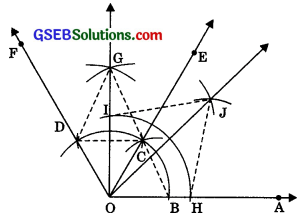(3) Taking C as center and with the same radius as before, draw an arc intersecting the arc drawn in step 1, say at D.
(4) Draw the ray OE passing through C. Then ∠EOA = 600.
(5) Draw the ray OF passing through D. Then ∠FOE = 600.
(6) Next, taking C and D as centers and with the radius more than CD, draw arcs to intersect each other, say at G.
(7) Draw the ray OG. This ray OG is the bisector of the angle ∠FOE, i.e.,
∠FOG = ∠EOG = $$\frac {1}{2}$$∠FOE = $$\frac {1}{2}$$(60°) = 30°.
Thus, ∠GOA = ∠GOE + ∠EOA = 30° + 60° = 90°.
Justification:
(i) Join BC,
Then, OC = OB = BC (By construction)
∴ ΔCOB is an equilateral triangle.
∴ ∠COB = 600
∴ ∠EOA = 60°(ii) Join CD,
Then, OD = OC = CD (By construction)
∴ ΔDOC is an equilateral triangle.
∴ ∠DOC = 600
∴ ∠FOE = 60°

(iii) Join CG and DG, In ΔODG and ΔOCG,
OD = OC [Radii of same arc]
DG = CG [Ares of equal radii]
OG = OG [Common]
∴ ΔODG = ΔOCG [SSS rulel]
∴ ∠DOG = ∠COG [CPCTI]
∴ ∠FOG = ∠ EOG = $$\frac {1}{2}$$ ∠FOE
= $$\frac {1}{2}$$(60) = 30
Thus, ∠GOA = ∠GOE + ∠EOA
= 30° + 60° = 90°.

Question 2.
Construct an angle of 450 at the initial point of a given ray and justify the construction.
Solution:
Given: A ray OA.
Required: To construct an angle of 450 at O and justify the construction. Steps of construction:
(1) Taking O as a centre and the same radius, draw an arc of a circle, which bisects OA, say at a point B.
(2) Taking B as centre and with the same radius as before, draw an arc intersecting the previously drawn arc, say at a point C.
(3) Taking C as centre and with the same radius as before, draw an arc intersecting the arc drawn in step 1, say at D.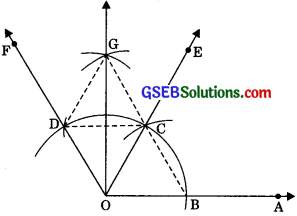(4) Draw the ray OE passing through C. Then, ∠EOA = 600.
(5) Draw the ray OF passing through D. Then, ∠FOE = 60°.
(6) Next, taking C and D as centres and with radius more than CD, draw arcs to intersect each other, say at G.
(7) Draw the ray OG. This ray OG is the bisector of the angle FOE, i.e.,
∠FOG = ∠EOG = $$\frac {1}{2}$$ ∠FOE = $$\frac {1}{2}$$ (60°) = 30°
Thus, ∠GOA = ∠GOE + ∠EOA
= 30° + 60° = 90°.
(8) Now, taking O as a centre and any radius, draw an arc to intersect the ray OA and OG, say at H and I respectively.
(9) Next, taking H and I as centres and with the radius more than $$\frac {1}{2}$$HI, draw arcs to intersect each other, say at J.
(10) Draw the ray OJ. This ray OJ is the required bisector of the angle GOA. Thus,
∠GOJ = ∠AOJ = $$\frac {1}{2}$$ ∠GOA
= $$\frac {1}{2}$$ (90°) = 45°Justification:
(i) Join BC,
Then, OC = OB = BC (By construction)
∴ ΔCOB is an equilateral triangle.
∴ ∠COB = 60°
∴ ∠EOA = 60°

(ii) Join CD,
Then, OD = OC = CD(By construction)
∴ DOC is an equilateral triangle.
∴ ∠DOC= 60°
∴ ∠FOE = 60°

(iii) Join CG and DG,
In ∠ODG and ∠OCG,
OD = OC (Radii of the same arc]
DG = CG [Arcs of equal radii]
OG = OG [Common]
∴ ΔODG = ΔOCG (SSS rule]
∴ ∠DOG = ∠COG [cpct]
∴ ∠FOG = ∠EOG = $$\frac {1}{2}$$ ∠FOE
= $$\frac {1}{2}$$ (60°) = 30°
Thus, ∠GOA =∠GOE + ∠EOA
= 30° + 60° = 90°

(iv) Join HJ and IJ,
In ΔOIJ and ΔOHJ,
OI = OH [Radii of the same arc]
IJ = HJ (Arcs of equal radlii]
OJ = OJ [Common]
∴ ΔOIJ = ΔOHJ [SSS rule]
∴ ∠IOJ = ∠HOJ [ICPCT]
∴ ∠AOJ = ∠GOJ = $$\frac {1}{2}$$ ∠GOA
= $$\frac {1}{2}$$ (90°) = 45°Question 3.
Construct the angles of the following measurements:
(i) 30°
(ii) 22$$\frac {1}{2}$$°
(iii) 15°
Solution:
(i) 30
Given: A ray OA.
Required: To construct an angle of 300 at O.
Steps of construction:
(1) Taking O as a centre and the same radius, draw an arc of a circle, which bisects OA, say at a point B.
i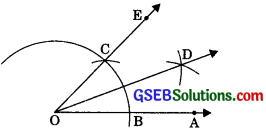(2) Taking B as centre and with the same radius as before, draw an arc intersecting the previously drawn arc, say at a point C.
(3) Draw the ray 0E passing through C. Then, ∠EOA = 60°.
(4) Taking B and C as centres and with the radius more than $$\frac {1}{2}$$ BC, draw arcs to intersect each other, say at D.
(5) Draw the ray OD. This ray OD is the bisector of the angle EOA i.e.,
∠EOD = ∠AOD = $$\frac {1}{2}$$ ∠EOA
= $$\frac {1}{2}$$(60°) = 30°.

(ii) 22$$\frac {1}{2}$$°
Given: A ray OA.
Required: To construct an angle of 22 at O.
Steps of construction:
(1) Taking O as a centre and the same radius, draw an arc of a circle, which intersects OA, say at a point B.
(2) Taking B as centre and with the same radius as before, draw an arc intersecting the previously drawn arc, say at a point C.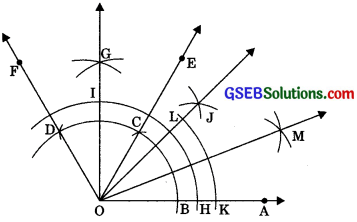(3) Taking C as centre and with the same radius as before, draw an arc intersecting the arc drawn in step 1, say at D.
(4) Draw the ray 0E passing through C. Then, ∠EOA = 600.
(5) Draw the ray OF passing through D. Then, ∠FOE = 60°.
(6) Next, taking C and D as a centre and with radius more than CD, draw arcs to intersect each other, say at G.
(7) Draw the ray OG. This ray OG is the bisector of the angle FOE, i.e.,
∠FOG = ∠EOG = $$\frac {1}{2}$$∠FOE
= $$\frac {1}{2}$$(60°) = 30°
Thus, ∠GOA = ∠GOE + ∠EOA
= 30° + 60° = 90°
(8) Now, taking O as the centre and any radius, draw an arc to intersect the ray OA and OG, say at H and I respectively.
(9) Next, taking H and I as centres and with the radius more than – HI, draw arcs to intersect each other, say at J.
(10) Draw the ray OJ. This ray OJ is the required bisector of the angle GOA. i.e.,
∠GOJ = ∠AOJ = $$\frac {1}{2}$$ ∠GOA
= $$\frac {1}{2}$$ (90°) = 45°
(11) Now, taking O as the centre and any radius, draw an arc to intersect the rays OA and OJ, say at K and L respectively.
(12) Next, taking K and L as centres and with the radius more than $$\frac {1}{2}$$ KL, draw arcs to intersect each other, say at M.
(13) Draw the ray OM. This ray OM is the bisector of the angle ∠AOJ, i.e., ∠JOM = ∠AOM
= $$\frac {1}{2}$$ ∠AOJ = $$\frac {1}{2}$$(45°) = 22 $$\frac {1}{2}$$°(iii) 15°
Given: A ray OA.
Required: To construct an angle of 15° atO.
Steps of construction:
(1) Taking O as a centre and same radius, draw an arc of a circle, which intersects OA, say at a point B.
(2) Taking B as centre and with the same radius as before, draw an arc intersecting the previously drawn arc, say at a point C.(3) Draw the ray 0E passing through C. Then, ∠EOA = 60°.
(4) Now, taking B and C as a centre and with radius more than $$\frac {1}{2}$$ BC, draw arcs to intersect each other, say at D.
(5) Draw the ray OD intersecting the arc drawn in step 1 at F. This ray OD is the bisector of the angle EOD, i.e., ∠EOD = ∠AOD
= $$\frac {1}{2}$$ ∠E0A = $$\frac {1}{2}$$ (60°) = 30°.
(6) Now, taking B and F as centres and with the radius more than $$\frac {1}{2}$$ BF, draw arcs to intersect each other, say at G.
(7) Draw the ray OG. This ray OG is the bisec tor of the angle AOD, i.e., ∠DOG = ∠AOG = $$\frac {1}{2}$$ ∠A0D
= $$\frac {1}{2}$$ (30°) = 15.Question 4.
Construct the following angles and verify by measuring them by a protractor:
(i) 750
(ii) 105°
(iii) 135°
Solution:
(i) 750
Given: A ray OA.
Required: To construct an angle of 750 at O.
Steps of construction:
(1) Taking O as a centre and same radius, draw an arc of a circle, which intersects OA, say at a point B.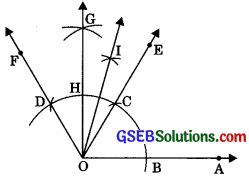(2) Taking B as centre and with the same radius as before, draw an arc intersecting the previously drawn arc, say at a point C.
(3) Taking C as centre and with the same radius as before, draw an arc intersecting the arc drawn in step 1, say at D.
(4) Draw the ray OE passing through C. Then, ∠EOA = 60°.
(5) Draw the ray OF passing through D. Then, ∠FOE = 60°.
(6) Next, taking C and D as centres and with the radius more than $$\frac {1}{2}$$CD, draw arcs to intersect each other, say at G.
(7) Draw the ray OG intersecting the arc of; step I at H. This ray OG is the bisector of the angle FOE,
i.e., ∠FOG = ∠EOG = $$\frac {1}{2}$$ ∠FOE
= $$\frac {1}{2}$$ (60°) = 30°.
(8) Next, taking C and H as centres and with the radius more than $$\frac {1}{2}$$ CH, draw arcs to intersect each other, say at I.
(9) Draw ray OI. This ray OI is the required bisector of the angle GOE. i.e.,
∠GOI = ∠EOI = $$\frac {1}{2}$$ ∠GOE = $$\frac {1}{2}$$ (30°) = 15°.
Thus, ∠IOA = ∠TOE + ∠EOA = 15° + 60° = 75°
On measuring the ∠IOA by protractor, we find that ∠IOA = 75°. Thus, the construction is verified.

(ii) 105°
Given: A ray OA.
Required: To construct an angle of 105° at O.
Steps of construction:
(1) Taking O as a centre and same radius, draw an arc of a circle, which intersects OA, say at a point B.(2) Taking B as centre and with the same radius as before, draw an arc intersecting the previously drawn arc, say at a point C.
(3) Taking C as centre and with the same radius as before, draw an arc intersecting the arc drawn in step 1, say at D.
(4) Draw the ray OE passing through C. Then, ∠EOA = 60°.
(5) Draw the ray OF passing through D. Then, ∠FOE = 60°.
(6) Next, taking C and D as centres and with radius more than $$\frac {1}{2}$$ CD, draw arcs to intersect each other, say at G.
(7) Draw the ray OG intersecting the arc drawn in step 1 at H. This ray OG is the bisector of the angle FOE,
i.e., ∠FOG = ∠EOG = $$\frac {1}{2}$$ ∠FOE
= $$\frac {1}{2}$$ (60°) = 30°.
Thus, ∠GOA = ∠GOE + ∠EOA
= 30° + 60° = 90°.
(8) Next, taking H and D as centres and with the radius more than $$\frac {1}{2}$$ID, draw arcs to intersect each other, say at I
(9) Draw the ray OI. This ray OI is the bisector of the angle FOG.
i.e., ∠FOI = ∠IOG = $$\frac {1}{2}$$ I0G = $$\frac {1}{2}$$ (30°) = 15°.Thus, ∠IOA = ∠IOG + ∠GOA = 15 + 90° = 105°.
On measuring the ¿IOA by protractor, we find that ∠IOA = 105°. Thus, the construction is verified.

(iii) 135°
Given: A ray OA.
Required: To construct an angle of 135° atO.
Steps of construction:
(1) Produce AO to A’ to form ray OA’.
(2) Taking O as a centre and same radius, draw an arc of a circle, which bisects OA, say at a point B.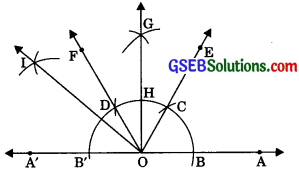(3) Taking B as centre and with the same radius as before, draw an arc intersecting the previously drawn arc, say at a point C.
(4) Taking C as centre and with the same radius as before, draw an arc intersecting the arc drawn in step 1, say at D.
(5) Draw the ray OE passing through C. Then, ∠EOA = 60°.
(6) Draw the ray OF passing through D. Then, ∠FOE = 60°.
(7) Next, taking C and D as centres and with radius more than $$\frac {1}{2}$$ CD, draw arcs to intersect each other, say at G.
(8) Draw the ray OG intersecting the arc drawn in step 1 at H. This ray OG is the bisector of the angle FOE, i.e.,
∠FOG = ∠EOG
= $$\frac {1}{2}$$ ∠FOE = $$\frac {1}{2}$$ (60°) = 30°
Thus, ∠GOA = ∠GOE + ∠EOA
= 30° + 60° = 90°.
∴ ∠B’OH = 90°.
(9) Next, taking B’ and H as centres and with the radius more than $$\frac {1}{2}$$ B’H, draw arcs to intersect each other, say at I.
(10) Draw the ray OG. This ray OI is the required bisector of the angle B’OD. i.e.,
∠B’OG = ∠GOG
= $$\frac {1}{2}$$ ∠B’OF = $$\frac {1}{2}$$ (90°) = 45°
Thus, ∠IOA = ∠IOG + ∠GOA = 45° + 90° = 135°.
On measuring the ∠IOA by protractor, we find that ∠IOA = 135°.
Thus, the construction is verified.Question 5.
Construct an equilateral triangle, given its side, and justify the construction.
Solution:
Given: Side (say 6 cm) of an equilateral triangle.
Required: To construct the equilateral triangle and justify the construction.
Steps of construction:
(1) Take a ray AX with initial point A. From AX cut off AB= 6cm.
(2) Taking A as centre and radius (= 6 cm), draw an arc of a circle, which intersects AX, say at a point B.(3) Taking B as centre and with the same radius as before, draw an arc intersecting the previously drawn arc, say at a point C.
(4) Draw the ray AE passing through C.
(5) Draw the ray BF passing through C. Then AABC is the required triangle with a given side 6 cm.
Justification:
AB = BC (By construction)
AB = AC (By construction)
∴ AB = BC = CA
∴ ∠ABC is an equilateral triangle.
∴ The construction is justified.